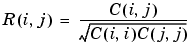MATLAB Function Referencecorrcoef

Correlation coefficients

Syntax

• ```R = corrcoef(X)
R = corrcoef(x,y)
[R,P]=corrcoef(...)
[R,P,RLO,RUP]=corrcoef(...)
[...]=corrcoef(...,'param1',val1,'param2',val2,...)
```

Description

```R = corrcoef(X) ``` returns a matrix `R` of correlation coefficients calculated from an input matrix `X` whose rows are observations and whose columns are variables. The matrix `R` `=` `corrcoef(X)` is related to the covariance matrix `C` `=` `cov(X)` by

•`corrcoef(X)` is the zeroth lag of the normalized covariance function, that is, the zeroth lag of `xcov(x,'coeff')` packed into a square array.

```R = corrcoef(x,y) ``` where `x` and `y` are column vectors is the same as `corrcoef([x y])`.

```[R,P]=corrcoef(...) ``` also returns `P`, a matrix of p-values for testing the hypothesis of no correlation. Each p-value is the probability of getting a correlation as large as the observed value by random chance, when the true correlation is zero. If `P(i,j)` is small, say less than `0.05`, then the correlation `R(i,j)` is significant.

```[R,P,RLO,RUP]=corrcoef(...) ``` also returns matrices `RLO` and `RUP`, of the same size as `R`, containing lower and upper bounds for a 95% confidence interval for each coefficient.

```[...]=corrcoef(...,'param1',val1,'param2',val2,...) ``` specifies additional parameters and their values. Valid parameters are the following.

 `'alpha'` A number between 0 and 1 to specify a confidence level of 100*(1 - `alpha`)%. Default is 0.05 for 95% confidence intervals. `'rows'` Either `'all'` (default) to use all rows, `'complete'` to use rows with no `NaN` values, or `'pairwise'` to compute `R(i,j)` using rows with no `NaN` values in either column `i` or `j`.

The p-value is computed by transforming the correlation to create a t statistic having `n`-2 degrees of freedom, where `n` is the number of rows of `X`. The confidence bounds are based on an asymptotic normal distribution of `0.5*log((1+R)/(1-R))`, with an approximate variance equal to `1/(n-3)`. These bounds are accurate for large samples when `X` has a multivariate normal distribution. The `'pairwise'` option can produce an `R` matrix that is not positive definite.

Examples

Generate random data having correlation between column 4 and the other columns.

• ```x = randn(30,4);     % Uncorrelated data
x(:,4) = sum(x,2);   % Introduce correlation.
[r,p] = corrcoef(x)  % Compute sample correlation and p-values.
[i,j] = find(p<0.05);  % Find significant correlations.
[i,j]                % Display their (row,col) indices.

r =
1.0000   -0.3566    0.1929    0.3457
-0.3566    1.0000   -0.1429    0.4461
0.1929   -0.1429    1.0000    0.5183
0.3457    0.4461    0.5183    1.0000

p =
1.0000    0.0531    0.3072    0.0613
0.0531    1.0000    0.4511    0.0135
0.3072    0.4511    1.0000    0.0033
0.0613    0.0135    0.0033    1.0000

ans =
4     2
4     3
2     4
3     4
```

See Also

`cov`, `mean`, `median`, `std`, `var`

`xcorr`, `xcov` in the Signal Processing Toolbox

© 1994-2005 The MathWorks, Inc.